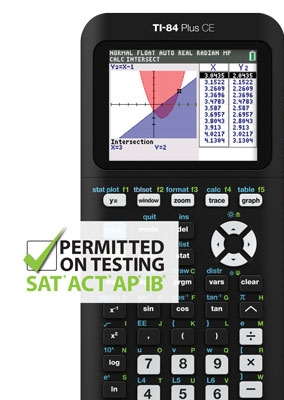Goldwise Development Limited# TI-84 Plus CEKey Features

30% lighter and thinner than earlier generation TI-84 Plus models

Vibrant backlit color screen

TI Rechargeable Battery

Pre-loaded Apps and Images

MathPrint™ functionality

EXAM ACCEPTANCE

The TI-84 Plus CE is approved for use on the following exams:

PSAT**, SAT**, and ACT® college entrance exams

AP** Exams that allow or require a graphing calculator

Praxis™ tests that allow or require a graphing calculator

Approved for use on the IB exam

Built-in Functionality

• Add images to graph background
• 15 colors for function graphing
• Change style and color of axes and grids on graphs
• Catalog Syntax Help
• Alphabetical CATALOG of all TI calculator operations in one menu
• Advanced functions accessed through pull-down display menus
• Real and complex numbers calculated to 14-digit accuracy and displayed with 10 digits plus a 2-digit exponent
• Graphs 10 rectangular functions, 6 parametric expressions, 6 polar expressions, and 3 recursively-defined sequences
• Up to 10 graphing functions defined, saved, graphed and analyzed at one time
• Sequence graphing mode shows time series plot, cobweb/stair-step plot and phase plots
• User-defined list names. Lists store up to 999 elements
• 17 interactive zoom features
• Numeric evaluations given in table format for all graphing modes
• Interactive analysis of function values, roots, maximums, minimums, integrals and derivatives
• 8 different graph styles for differentiating the look of each graph drawn
• Horizontal and vertical split- screen options
• View graph and table side-by-side
• Stores up to 10 - 50x50 matrices
• Matrix operations including inverse, determinant, transpose, augment, reduced row echelon form and elementary row operations. Convert matrices to lists and vice-versa
• List-based one- and two-variable statistical analysis, including logistic, sinusoidal, median-median, linear, logarithmic, exponential, power, quadratic polynomial, cubic polynomial, and quartic polynomial regression models
• 3 statistical plot definitions for scatter plots, xy-line plots, histograms, regular and modified box-and-whisker plots, and normal probability plots
• Advanced statistics features including 10 hypothesis testing functions, 7 confidence interval functions and one-way analysis of variance
• 15 probability distribution functions including Normal, Student-t, Chi-square, Binomial, and Poisson
• Business functions including Time-Value-of-Money (TVM), cash flows, and amortization. Full screen interactive editor for solving TVM problems
• Interactive equation solver editor allows solving numerically for different variables in an equation
• 11 Regression calculations

*Use TI Connect™ CE Software to send files, take screen captures, create and edit TI Basic programs

**SAT & AP are registered trademarks of the College Board, which was not involved in the production of and does not endorse this product. ACT is a registered trademark of ACT, Inc., which does not endorse this product. Policies subject to change. Visit www.collegeboard.com and www.act.org